# A mass oscillates displaying the Simple Harmonic Motion

• Slides: 8
Download presentationA mass oscillates displaying the Simple Harmonic Motion plotted in the position vs time graph at right. 1) Which of the velocity vs time graphs best represent its motion? 2) 3) 4)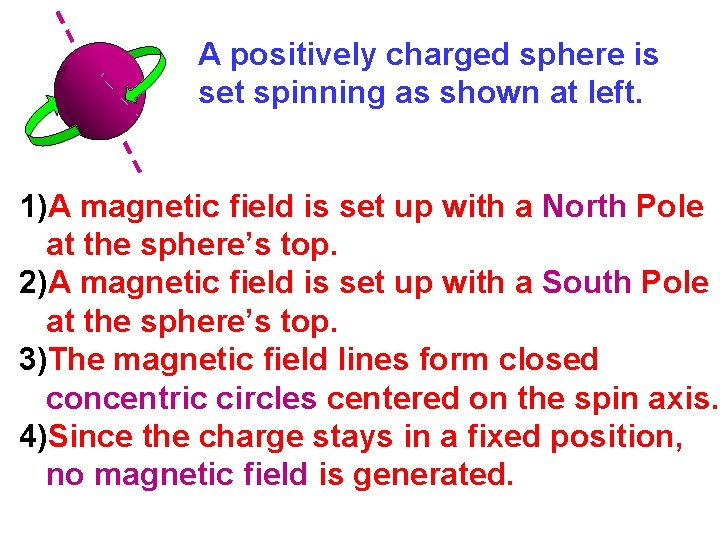A positively charged sphere is set spinning as shown at left. 1)A magnetic field is set up with a North Pole at the sphere’s top. 2)A magnetic field is set up with a South Pole at the sphere’s top. 3)The magnetic field lines form closed concentric circles centered on the spin axis. 4)Since the charge stays in a fixed position, no magnetic field is generated.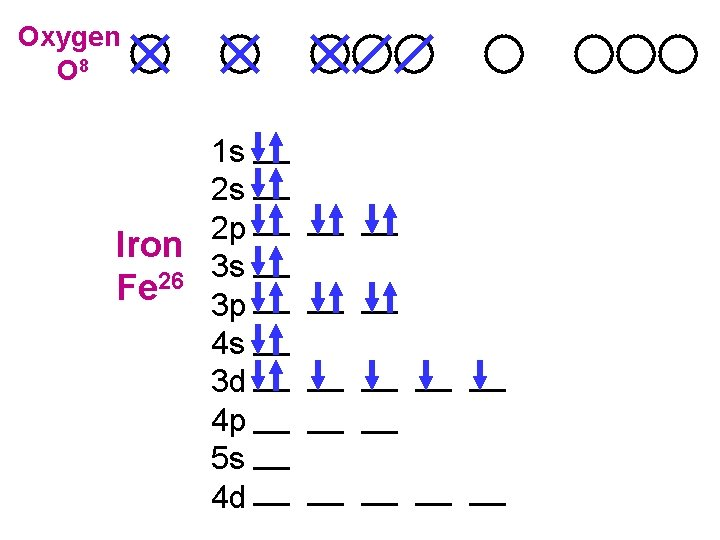Oxygen O 8 1 s 2 s 2 p Iron 3 s 26 Fe 3 p 4 s 3 d 4 p 5 s 4 dIf a N pole moves towards a loop, both in the plane of the page, in what direction is the induced current? 1) clockwise S 2) counter-clockwise 3) no induced current N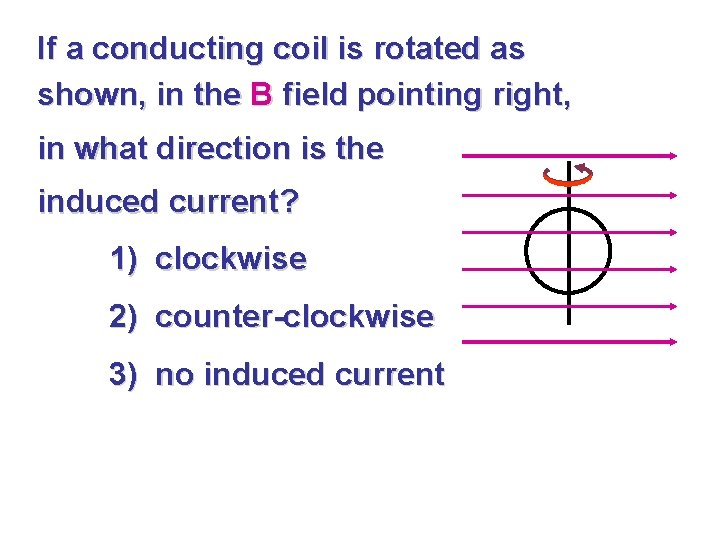If a conducting coil is rotated as shown, in the B field pointing right, in what direction is the induced current? 1) clockwise 2) counter-clockwise 3) no induced currentWire #1 (length L) forms a 1 -turn loop, and a bar magnet is dropped through. Wire #2 (length 2 L) forms a 2 -turn loop, #1 the same magnet is dropped through. #2 Compare the induced EMF’s for both. 1) 1< 2 2) 1 = 2 3) 1 > 2 Compare the magnitude of the induced currents in these two cases. 1) I 1 < I 2 2) I 1 = I 2 3) I 1 > I 2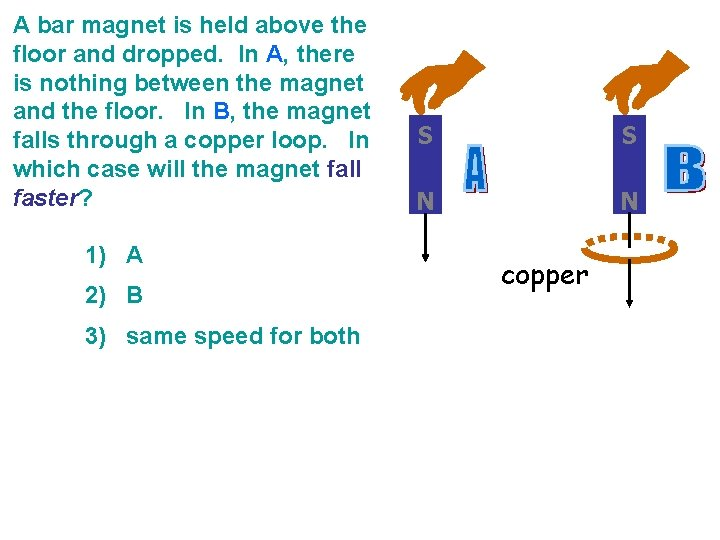A bar magnet is held above the floor and dropped. In A, there is nothing between the magnet and the floor. In B, the magnet falls through a copper loop. In which case will the magnet fall faster? 1) A 2) B 3) same speed for both S S N N copper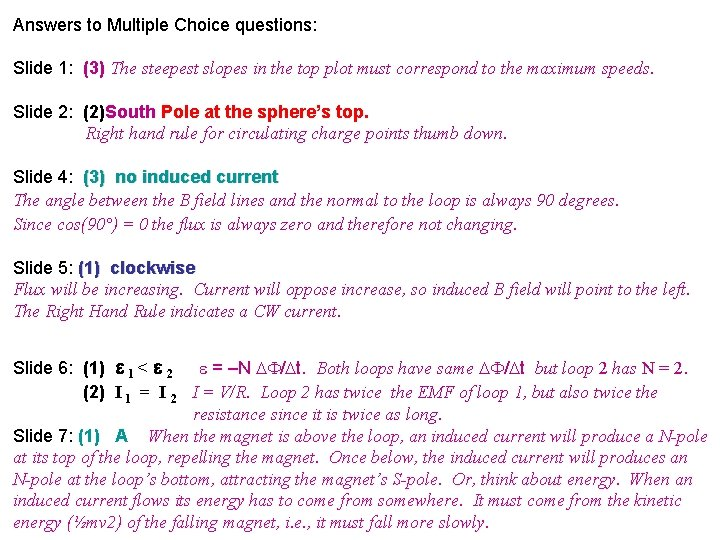Answers to Multiple Choice questions: Slide 1: (3) The steepest slopes in the top plot must correspond to the maximum speeds. Slide 2: (2)South Pole at the sphere’s top. Right hand rule for circulating charge points thumb down. Slide 4: (3) no induced current The angle between the B field lines and the normal to the loop is always 90 degrees. Since cos(90°) = 0 the flux is always zero and therefore not changing. Slide 5: (1) clockwise Flux will be increasing. Current will oppose increase, so induced B field will point to the left. The Right Hand Rule indicates a CW current. Slide 6: (1) 1 < 2 = –N / t. Both loops have same / t but loop 2 has N = 2. (2) I 1 = I 2 I = V/R. Loop 2 has twice the EMF of loop 1, but also twice the resistance since it is twice as long. Slide 7: (1) A When the magnet is above the loop, an induced current will produce a N-pole at its top of the loop, repelling the magnet. Once below, the induced current will produces an N-pole at the loop’s bottom, attracting the magnet’s S-pole. Or, think about energy. When an induced current flows its energy has to come from somewhere. It must come from the kinetic energy (½mv 2) of the falling magnet, i. e. , it must fall more slowly.